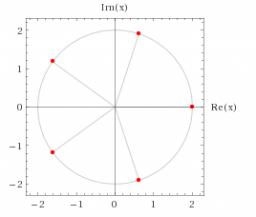# Equation: 3726

Determine the real root of the equation: x-3: x-8 = 32

x =  2

### Step-by-step explanation:Did you find an error or inaccuracy? Feel free to write us. Thank you!

Tips for related online calculators
Do you have a linear equation or system of equations and looking for its solution? Or do you have a quadratic equation?
Try our complex numbers calculator.